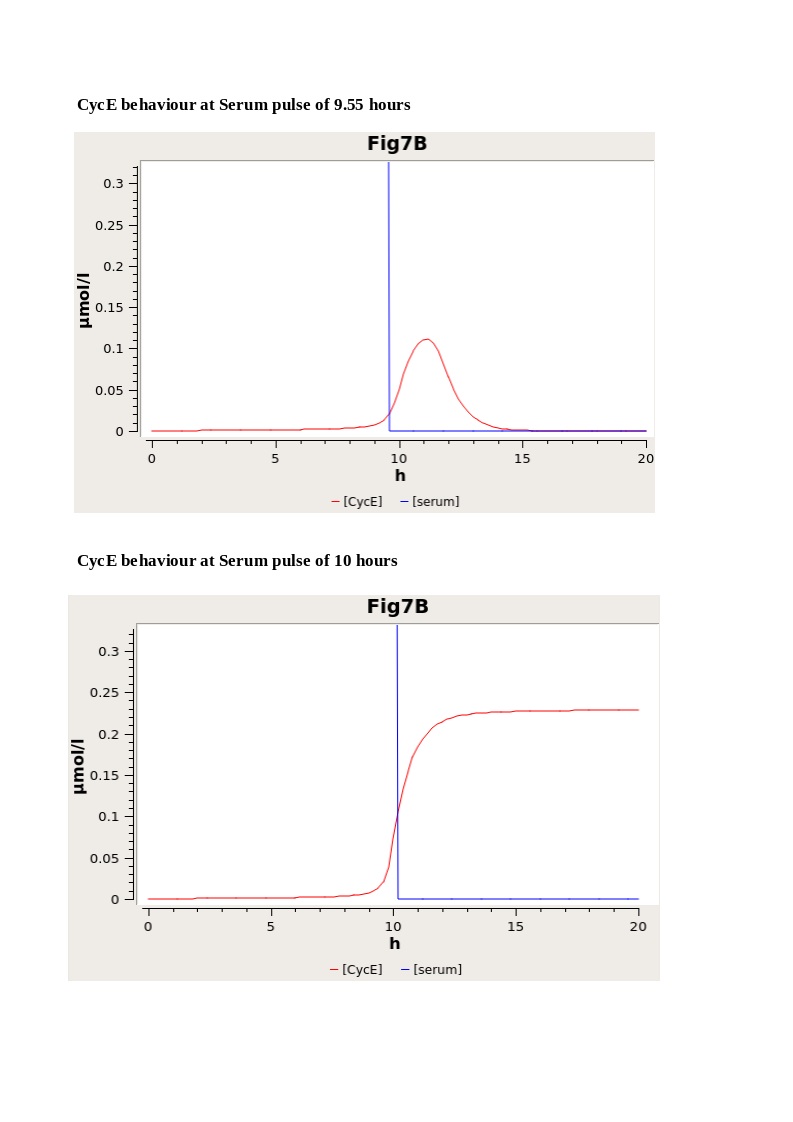## Schwarz2018-Cdk Activity Threshold Determines Passage through the Restriction PointModel Identifier
BIOMD0000000918
Short description
At the restriction point (R), mammalian cells irreversibly commit to divide. R has been viewed as a point in G1 that is passed when growth factor signaling initiates a positive feedback loop of Cdk activity. However, recent studies have cast doubt on this model by claiming R occurs prior to positive feedback activation in G1 or even before completion of the previous cell cycle. Here we reconcile these results and show that whereas many commonly used cell lines do not exhibit a G1 R, primary fibroblasts have a G1 R that is defined by a precise Cdk activity threshold and the activation of cell-cycle-dependent transcription. A simple threshold model, based solely on Cdk activity, predicted with more than 95% accuracy whether individual cells had passed R. That a single measurement accurately predicted cell fate shows that the state of complex regulatory networks can be assessed using a few critical protein activities.
Format
SBML (L2V4)
Related Publication
• A Precise Cdk Activity Threshold Determines Passage through the Restriction Point.• Schwarz C, Johnson A, Kõivomägi M, Zatulovskiy E, Kravitz CJ, Doncic A, Skotheim JM
• Molecular cell , 1/ 2018 , Volume 69 , Issue 2 , pages: 253-264.e5 , PubMed ID: 29351845
• Department of Biology, Stanford University, Stanford, CA 94305, USA.
• At the restriction point (R), mammalian cells irreversibly commit to divide. R has been viewed as a point in G1 that is passed when growth factor signaling initiates a positive feedback loop of Cdk activity. However, recent studies have cast doubt on this model by claiming R occurs prior to positive feedback activation in G1 or even before completion of the previous cell cycle. Here we reconcile these results and show that whereas many commonly used cell lines do not exhibit a G1 R, primary fibroblasts have a G1 R that is defined by a precise Cdk activity threshold and the activation of cell-cycle-dependent transcription. A simple threshold model, based solely on Cdk activity, predicted with more than 95% accuracy whether individual cells had passed R. That a single measurement accurately predicted cell fate shows that the state of complex regulatory networks can be assessed using a few critical protein activities.
Contributors

hasProperty
Mathematical Modelling Ontology Ordinary differential equation model
C18689
occursIn
hasTaxon
isDescribedBy
is

Curation status
Curated

Modelling approach(es)

Tags
Name Description Size Actions

### Model files

Schwarz2018.xml SBML L2V4 file Schwarz2018-Cdk Activity Threshold Determines Passage through the Restriction Point_ Curated 74.22 KB Preview | Download

Schwarz2018.sedml sed-ml L1V2_Schwarz2018-Cdk Activity Threshold Determines Passage through the Restriction Point_Curated 2.17 KB Preview | Download
Schwarz2018.cps COPASI version 4.27 (Build 217) Schwarz2018-Cdk Activity Threshold Determines Passage through the Restriction Point_ Curated 119.54 KB Preview | Download
Schwarz2018_orignal.xml SBML L2V4 file Schwarz2018-Cdk Activity Threshold Determines Passage through the Restriction Point_Original File 93.57 KB Preview | Download
• Model originally submitted by : Ahmad Zyoud
• Submitted: 05-Mar-2020 12:23:05
##### Revisions
Legends
: Variable used inside SBML models

Species
Species Initial Concentration/Amount
Myc

C18538
0.0 μmol
E2F

C129647
0.0 μmol
CycD

C104194
0.0 μmol
CycE

C104197
0.0 μmol
Phosphorylated Rb

0016708; phosphorylated
0.0 μmol
Rb

0016708
55.0 μmol
Rb E2F complex

Rb-E2F complex
0.0 μmol
Reactions
Reactions Rate Parameters
Cell*(kM*Myc/(KS+serum)-dM*Myc)

Cell*(kM*Myc/(KS+serum)-dM*Myc)
kM = 1.0; dM = 0.7; KS = 0.5
Cell*(((kE*(kpfb+Myc/(KM+Myc))*E2F/(KE+E2F)+kb*Myc/(KM+Myc)+kP1*CycD*Rb_E2F_complex/(KCD+Rb_E2F_complex)+kP2*CycE*Rb_E2F_complex/(KCE+Rb_E2F_complex))-dE*E2F)-kRE*Rb*E2F)

Cell*(((kE*(kpfb+Myc/(KM+Myc))*E2F/(KE+E2F)+kb*Myc/(KM+Myc)+kP1*CycD*Rb_E2F_complex/(KCD+Rb_E2F_complex)+kP2*CycE*Rb_E2F_complex/(KCE+Rb_E2F_complex))-dE*E2F)-kRE*Rb*E2F)
kP1 = 18.0; dE = 0.25; kb = 0.003; kpfb = 4.0; kP2 = 18.0; KCD = 0.92; KCE = 0.92; kE = 0.4; KM = 0.15; KE = 0.15; kRE = 180.0
Cell*((kCD*Myc/(KM+Myc)+kCDS*serum/(KS+serum))-dCD*CycD)

Cell*((kCD*Myc/(KM+Myc)+kCDS*serum/(KS+serum))-dCD*CycD)
kCD = 0.03; KM = 0.15; kCDS = 0.45; dCD = 1.5; KS = 0.5
Cell*(kCE*E2F/(KE+E2F)-dCE*CycE)

Cell*(kCE*E2F/(KE+E2F)-dCE*CycE)
kCE = 0.35; dCE = 1.5; KE = 0.15
Cell*((kP1*CycD*Rb/(KCD+Rb)+kP2*CycE*Rb/(KCE+Rb_E2F_complex)+kP1*CycD*Rb_E2F_complex/(KCD+Rb_E2F_complex)+kP2*CycE*Rb_E2F_complex/(KCE+Rb_E2F_complex))-dRP*Phosphorylated_Rb)

Cell*((kP1*CycD*Rb/(KCD+Rb)+kP2*CycE*Rb/(KCE+Rb_E2F_complex)+kP1*CycD*Rb_E2F_complex/(KCD+Rb_E2F_complex)+kP2*CycE*Rb_E2F_complex/(KCE+Rb_E2F_complex))-dRP*Phosphorylated_Rb)
kP1 = 18.0; kP2 = 18.0; dRP = 0.06; KCD = 0.92; KCE = 0.92
Cell*(((((kR+kDP*Phosphorylated_Rb/(KRP+Phosphorylated_Rb))-kRE*Rb*E2F)-kP1*CycD*Rb/(KCD+Rb))-kP2*CycE*Rb/(KCE+Rb))-dR*Rb)

Cell*(((((kR+kDP*Phosphorylated_Rb/(KRP+Phosphorylated_Rb))-kRE*Rb*E2F)-kP1*CycD*Rb/(KCD+Rb))-kP2*CycE*Rb/(KCE+Rb))-dR*Rb)
kR = 0.18; kP1 = 18.0; kP2 = 18.0; KCD = 0.92; KCE = 0.92; kDP = 3.6; dR = 0.06; kRE = 180.0; KRP = 0.01
Cell*(((kRE-kP1*CycD*Rb_E2F_complex/(KCD+Rb_E2F_complex))+kP2*CycE*Rb_E2F_complex/(KCE+Rb_E2F_complex))-dRE*Rb_E2F_complex)

Cell*(((kRE-kP1*CycD*Rb_E2F_complex/(KCD+Rb_E2F_complex))+kP2*CycE*Rb_E2F_complex/(KCE+Rb_E2F_complex))-dRE*Rb_E2F_complex)
kP1 = 18.0; kP2 = 18.0; dRE = 0.03; KCD = 0.92; KCE = 0.92; kRE = 180.0Curator's comment:
(added: 05 Mar 2020, 12:22:26, updated: 05 Mar 2020, 12:22:26)
To be able to reproduce a similar simulation for figure 7B, The initial value of the Rb was changed into 55uM rather than 0.55uM, Also the base serum pulse was changed into 0 However, the reproduced figure is not an exact replica of the 7B figure mentioned in the paper, the value of the y-axis doesn't fit ( much lower in the model)- as its been explained by the authors- because the model puts out cyclin E concentration which it has re-scaled to Cdk activity.